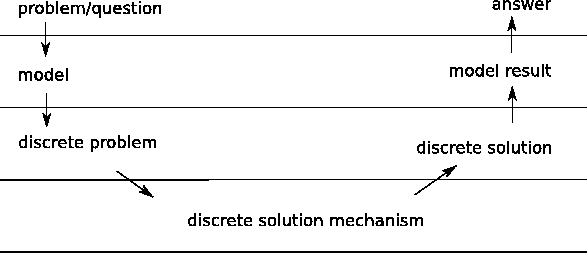# 1. 1 Classification of Simulation Processes

There exists a vast number of computer programs as well as programming frameworks which can be used in order to perform computer simulation. Programs use different kinds of approaches, solve different physical problems, various types of mathematical processes, and use a large variety of available algorithms and data structures.

The first step towards a rigorous specification and classification for the different kinds of programs used for computer aided engineering is to provide a layer model of abstract steps which are performed in a computer simulation. It is not necessary to make use of all of the layers provided in this layer model for all situations. In this section an approach towards a layered model is presented, which provides a clear separation of different steps which have to be performed, when designing a simulation program.

Another aspect of this model is to share workload within a group of scientists who have different expertise in various kinds of scientific computing. The reason for this separation using clearly defined interfaces is to establish a concise method of interaction between different scientists. Even though the mutual understanding is often very poor, the use of such interfaces enables scientists to establish proper computational methods.

For one person - in contrast - scientific computing is hardly overlookable and the development of methods in all different fields involved in the simulation is time consuming and does, therefore, not lead to optimal results. For this reason a sensible separation of competences has to be established in order to balance the workload.

The fields covered by scientific computing are modeling, discretization, algebraic methods, and program design, where program design can be considered as necessary for the implementation of methods of all other fields, which form the theoretical background. Program and data structural design is primarily required to define clear and concise interfaces between the single theoretical fields. For this reason a data structural interface is found for modeling, discretization and algebraic methods.

Modeling is required to find a mathematical model for a physical phenomenon of interest. It is typically carried out by physicists and engineers, who have a concrete question in mind. As an example for modeling the Schrödinger equation can be considered, which perfectly describes the behavior of particle systems with very in small scales under the influence of a potential.

Discretization is a general term for methods which make continuous problems solvable for the computer. Typical methods are the finite element method or the finite volume method. The solution of a linear or a nonlinear equation system as well as the solution of an eigenvalue problem is a typical representative of an algebraic method.

Even though the formulation of the problem in a programming language and the design of data structures which represent the mathematical structures used are relevant for the implementation of software, the layers show the abstract steps which have to be performed, but not how they have to be implemented.

Once these fields of scientific computing are separated, the layers can be easily defined. The original question is posed to the engineer or physicist who uses modeling in order to provide a continuously formulated problem which is discretized and solved by a computer aided mechanism. After the solution is obtained by the computer it is often checked for validity in a mathematical manner. This means that the solution fulfills the given mathematical equation. Furthermore the physical accuracy has to be checked, which means that the physical model is used within its boundaries of validity.

Most of the available models inherently have restrictions which have to be considered before the solution can be validated. In the following the main focus is put on the abstract methods rather than the implementation. Even though the use of computers is an essential aid when solving large equation systems and software is an essential means for making the problems concise and easy to formulate, the mathematical and physical problems can be also performed in an abstract manner or - in some cases - even by hand. The main focus is put on the separation of the single stages, where each of the stages can be simplified by the computer.

It has to be considered that all these mental steps can be carried out independently from each other. While some steps naturally induce a special treatment in the underlying layers, a premature restriction on some methods unnecessarily restricts the variety of possible solution approaches. Fig. 1.1 shows the single steps of the simulation as well as the layers of the classification.Subsections
Michael 2008-01-16